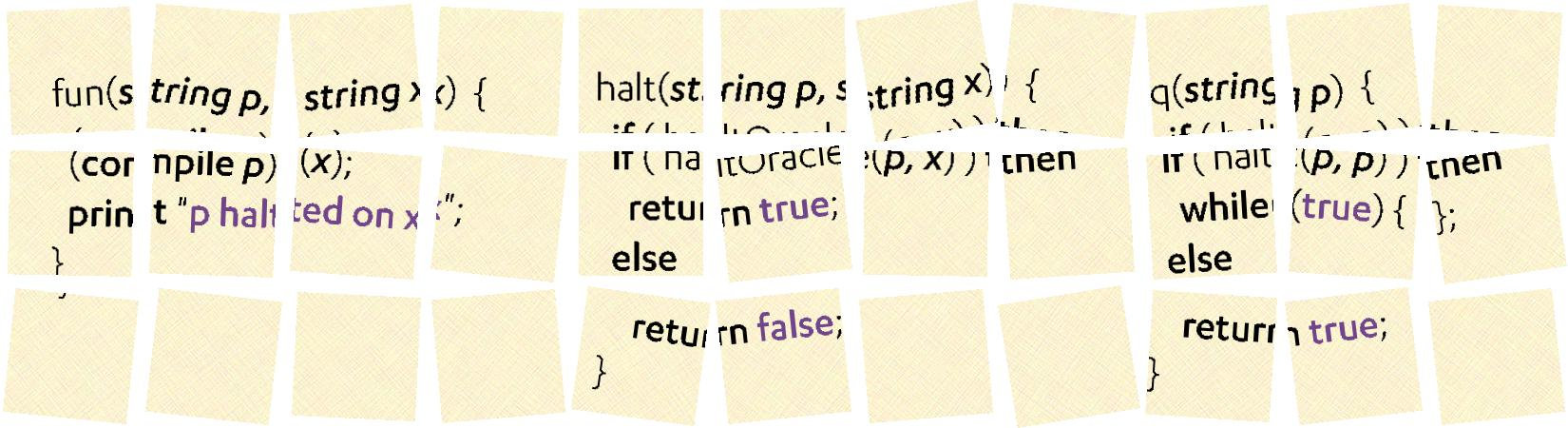# General malware detectors are impossibleIt is impossible to write a general purpose malware detector. Not hard, not difficult, impossible. The argument for the impossibility relies on building an odd program. We may not write such a program in practice, but it does arise as a combination of things we do write — things like Perl-like regular expressions and input parsers — and carefully crafted inputs.

The type of malware we often worry about is the type that alters the environment of the CPU. Programs that change data in the disk, that send information over the network, or that alter what is on our display. (I’m calling all the other hardware attached to the CPU its environment.) There is malware that just keeps the CPU busy without interacting with the environment, but those need an installer, which is malware of the environment changing type, so we only need to deal with those.

## The proof

Suppose that we come up with a class of interactions with the environment that we deem malicious and call $M$ the set of all programs with those properties. Things like exfiltrate our passwords, make our files unreadable, etc. A malware detector $v$ would be a program that determines if an input program $p$ belongs to the class of malware $M,$ that is, does $v(p)$ returns true. The claim is that there is no such $v.$

The reason is that if such a program existed, it would imply the solution of the halting problem, introduced and solved by Turing in his original paper on the computer. Imagine that we have checked that a program $q$ belongs to $M$ using our procedure $v.$ Now take a new program $r$ that does not alter the environment, so does not belong to $M,$ and returns nothing. Use it to create a new program $p$ that first executes $r$ and then executes $q.$ As $r$ does nothing to the environment nor does it change $q$ in any way, deciding whether $p$ belongs to $M$ or not rests on $r$ terminating or not. If it terminates, then $q$ executes making $p$ do exactly what $q$ does and therefore a member of $M,$ but if $r$ does not terminate, then $q$ never executes and $p$ is not a member of $M.$ That is, deciding if $p \in M$ requires knowing if $r$ halts, which Turing proved impossible in general.

## What matters is the shadow it casts

This impossibility result is just a fancy way of saying that it is hard to discover what a program does by just looking at it. This is intuitive to anyone that has tried to debug a program. It manifests itself in many other impossibility results of practical consequence. A similar argument would apply to a bug finding program, or even a more limited memory leak finding program. There are program analyzers that apply heuristics to find classes of bugs, but even a narrow class of bugs, such as buffer overflow from input, is hard to detect despite being a problem for over two decades.

These negative results do not imply that it is impossible to prove something about a program or a class of programs. Turing himself later introduced one of the basic techniques to prove program termination: Find a function that takes values on a lattice (positive integers are a good example); arrange it so that for each expression in the program the function decreases in value; and check that it happens regardless of the input values. Turing’s method has been elaborated by using many such functions, by using Ramsey’s theory and by introducing Galois theory to find better functions. These methods handle pratical programs with thousands of lines of code, but still suffer with complex numerics, elaborate data structures, and fancy bit operations. Attackers knowing that could make present the ghost of the halting problem by obfuscating their program using things that are hard for program analyzers.